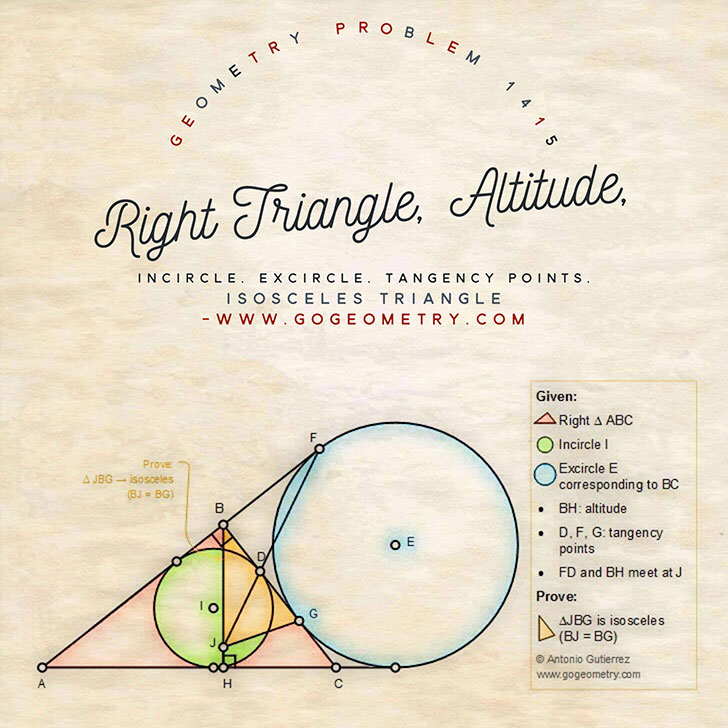# Geometry Problem 1415: Right Triangle, Altitude, Incircle, Excircle, Tangency Points, Isosceles Triangle

### Proposition

The figure below shows a right triangle ABC (angle B = 90 degree) with the altitude BH. The incircle I is tangent to BC at D. The excircle E corresponding to BC is tangent to BC at G and tangent to the extension of AB at F. FD extended and BH meet at J. Prove that the triangle JBG is an isosceles triangle (BJ = BG).### Geometric Art of Problem 1415: Sketching, Typography, using iPad